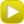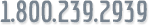Energy Cost Calculator

Enter the following information to calculate your estimated yearly and monthly energy costs.

 Hours per Day Days per Year Wattage per Fixture Number of Fixtures Fixture Type Cost per KwH 8 12 16 24 25 40 60 75 100 175 250 400 Incandescent Fluorescent HID .08 .10 .12 .14 .16 .18

\$0.00 / month

When operating on a photocell or timeclock the basic equation is:
(Hours per day) x (Days per year) x (Wattage per fixture) x (\$ Cost per kWh*) x 0.001 = ANNUAL COST
*
Total Monthly electric bill / kWh’s used = \$ Cost per kWh

The kilowatt-hour (kWh) = 1000 watt-hours;
One watt-hour is the amount of energy (usually electrical) expended by a one-watt load (e.g. light bulb) drawing power for one hour. [see Wikipedia]...